# The Universe of Discourse

Sat, 12 Dec 2015

This is page 22 of the Cosmic Call message. An explanation follows.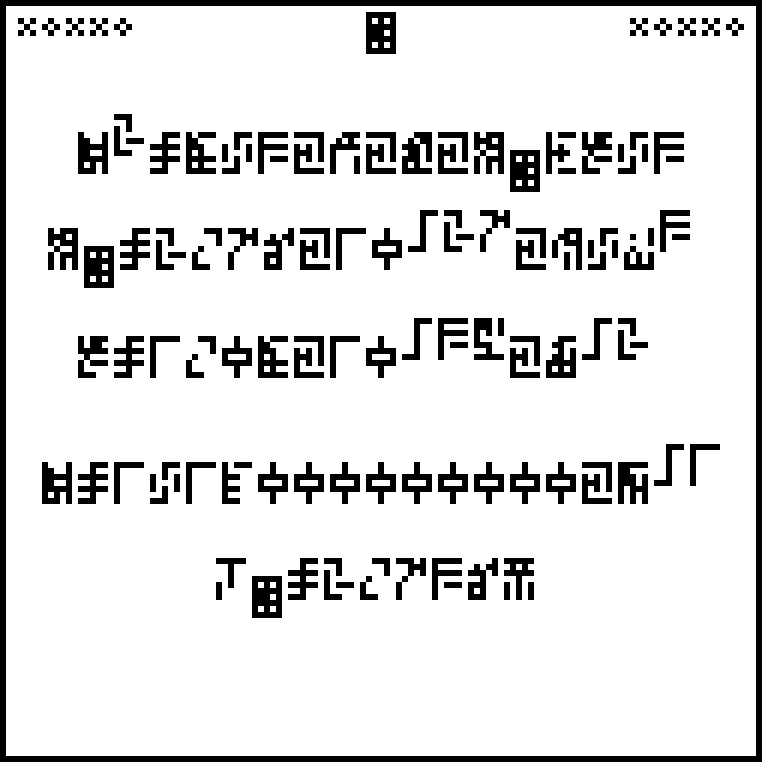The 10 digits are: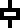0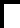1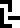2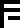3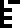4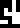5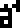6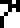7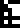8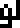9

This page discusses properties of the entire universe. It is labeled with a new glyph,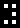, which denotes the universe or the cosmos. On this page I am on uncertain ground, because I know very little about cosmology. My explanation here could be completely wrong without my realizing it.

The page contains only five lines of text. In order, they state:

1. The Friedmann equation which is the current model for the expansion of the universe. This expansion is believed to be uniform everywhere, but even if it isn't, the recipients are so close by that they will see exactly the same expansion we do. If they have noticed the expansion, they may well have come to the same theoretical conclusions about it. The equation is:

$$H^2 = \frac{8\pi G}3\rho + \frac{\Lambda c^2 }3$$

where !!H!! is the Hubble parameter (which describes how quickly the universe is expanding), !!G!! is the universal gravitation constant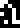(introduced on page 9), !!\rho!! is the density of the universe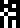(given on the next line), and !!\Lambda c^2!! (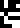) is one of the forms of the cosmological constant (given on the following line).

2. The average densityof the universe, given as !!2.76\times 10^{-27} \mathrm{kg} ~\mathrm{m}^{-3}!!. The “density” glyph would have been more at home with the other physics definitions of page 9, but it wasn't needed until now, and that page was full.

3. The cosmological constant !!\Lambda!! is about !!10^{-52} \mathrm{m}^{-2}!!. The related value given here, !!\Lambda c^2!!, is !!1.08\cdot 10^{-35} \mathrm{s}^{-2}!!.

4. The calculated value of the Hubble parameter !!H!!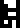is given here in the rather strange form !!\frac1{14000000000}\mathrm{year}^{-1}!!. The reason it is phrased this way is that (assuming that !!H!! were constant) !!\frac1H!! would be the age of the universe, approximately 14,000,000,000 years. So this line not only communicates our estimate for the current value of the Hubble parameter, it expresses it in units that may make clear our beliefs about the age of the universe. It is regrettable that this wasn't stated more explicitly, using the glyph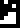that was already used for the age of the Earth on page 13. There was plenty of extra space, so perhaps the senders didn't think of it.

5. The average temperature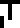of the universe, about 2.736 kelvins. This is based on measurements of the cosmic microwave background radiation, which is the same in every direction, so if the recipients have noticed it at all, they have seen the same CMB that we have.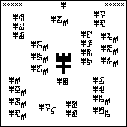The next article will discuss the final page, shown at right. (Click to enlarge.) Try to figure it out before then.